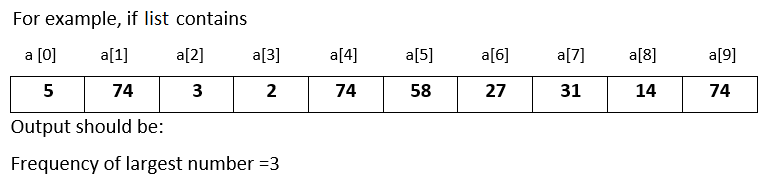# Input 10 numbers in integer list and find the frequency of the largest number in Python

###### Lists - Question 5

In this question, we will see how to input 10 numbers in integer list and find the frequency of the largest number in Python programming. To know more about lists click on the lists lesson.

Q5) Write a program in Python to input 10 numbers in integer list and find the frequency of the largest number.#### Program

``````ln=0
a=list()

print('Enter 10 numbers')
for i in range(10):
a.append(int(input()))

for i in range(10):
if i==0:
ln=a[i]

elif a[i]>ln:
ln=a[i]

print('Frequency of the Largest Number =',a.count(ln))``````

#### Output

```Enter 10 numbers
5
74
3
2
74
58
27
31
15
74
Frequency of the Largest Number = 3  ```